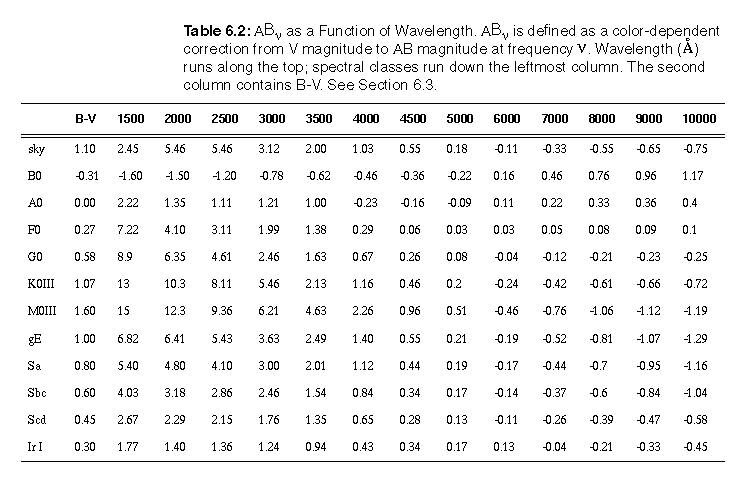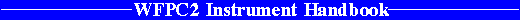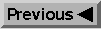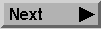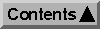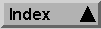System Throughput and SNR / Exposure Time Estimation

# 6.1 System Throughput

A decision on a suitable exposure time will require the combination of

• The overall spectral response of the system (Figure 2.4).

• The spectral transmission of the filters (Chapter 3 and Appendix A).

• The spectral energy distribution and spatial profile of the target.

• The point response function and pixel size of the instrument (Chapter 5).

• Criteria for specifying desirable charge levels.

When the transmissions of filters T(lambda) are combined with the overall system response Q(lambda), we obtain detective quantum efficiency plots (electrons-per-photon as a function of lambda) for each filter. These DQE plots link the output of the CCD to the photon flux at the input to an unobscured 2.4 m telescope.

The effective dimensionless Gaussian width is defined implicitly by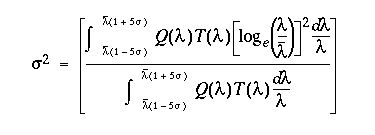The final two columns in Table 6.1 are defined as follows. In the next-to-last column me/sec is the zero-point magnitude for 1 e- s-1 (with AB_nu=0). The final column gives twfsky, which is the exposure time (in seconds) needed to make the sky noise equal to 5 e- RMS (i.e. ~read noise) in the WFC for a sky level of V=23.3 mag arcsec-2.

#### Table 6.1: System Efficiencies and Zeropoints.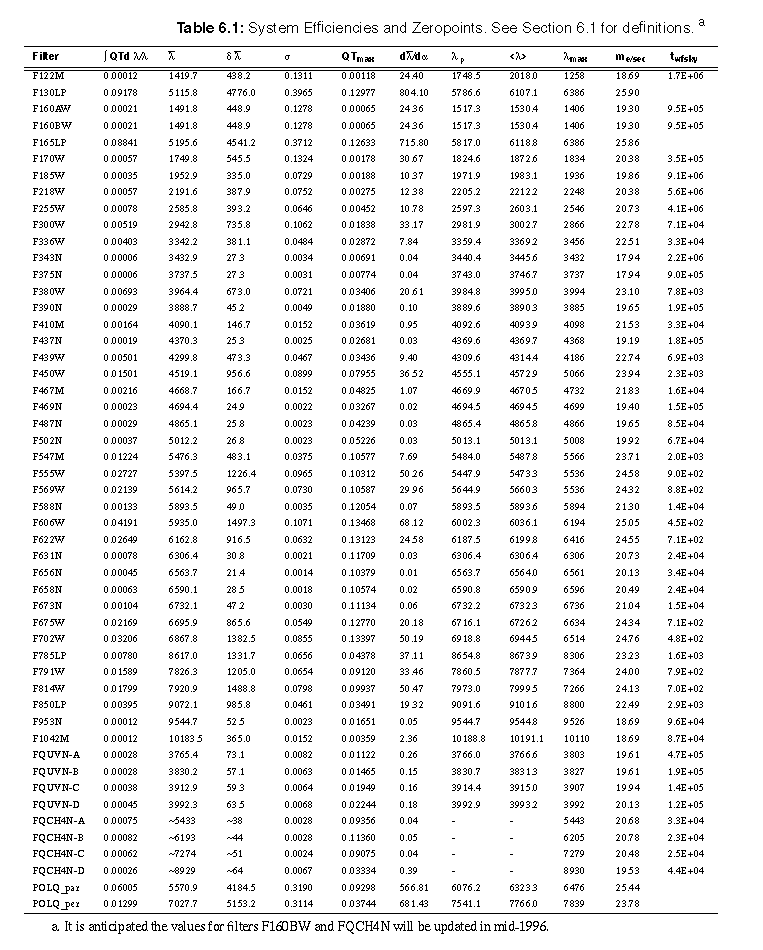#### Table 6.2: AB_nu as a Function of Wavelength.